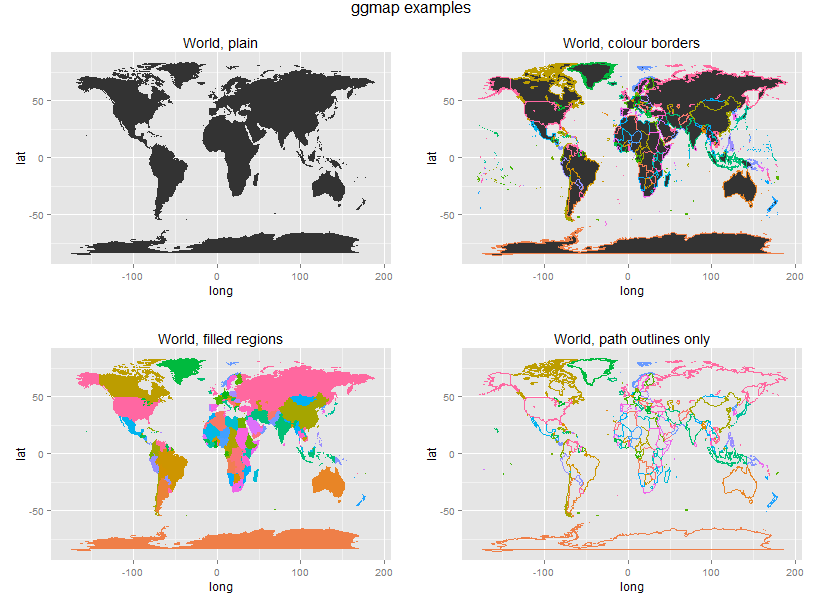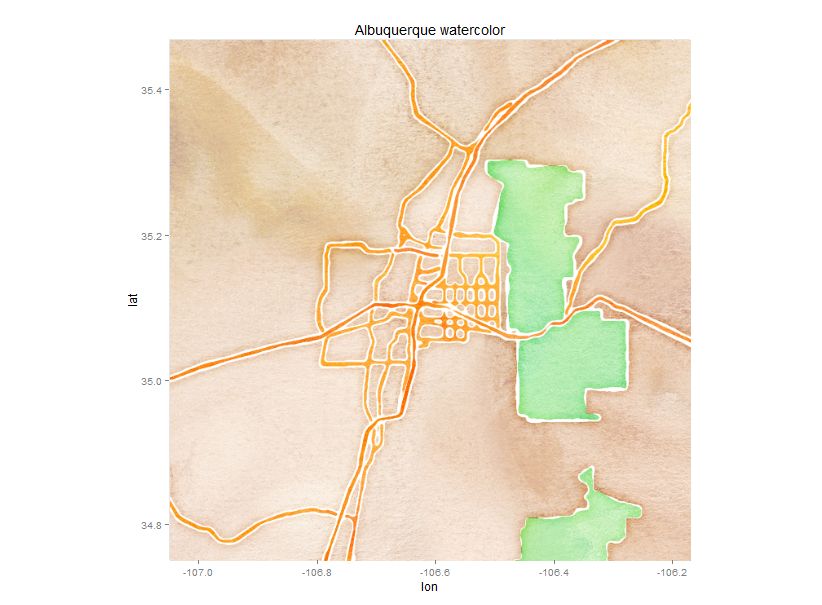# Drawing maps

## 1.1 rworldmap, World Map and countries

```library(rworldmap)   # start with the entire world newmap <- getMap(resolution = "low") plot(newmap, main = "World") # crop to the area desired (outside US) # (can use maps.google.com, right-click, drop lat/lon markers at corners) plot(newmap , xlim = c(-139.3, -58.8) # if you reverse these, the world gets flipped , ylim = c(13.5, 55.7) , asp = 1 # different aspect projections , main = "US from worldmap" )```## 1.2  ggmap, World Map and countries

```library(ggplot2)   map.world <- map_data(map = "world") # map = name of map provided by the maps package. # These include county, france, italy, nz, state, usa, world, world2. str(map.world) # how many regions length(unique(map.world\$region)) # how many group polygons (some regions have multiple parts) length(unique(map.world\$group)) p1 <- ggplot(map.world, aes(x = long, y = lat, group = group)) p1 <- p1 + geom_polygon() # fill areas p1 <- p1 + labs(title = "World, plain") #print(p1) p2 <- ggplot(map.world, aes(x = long, y = lat, group = group, colour = region)) p2 <- p2 + geom_polygon() # fill areas p2 <- p2 + theme(legend.position="none") # remove legend with fill colours p2 <- p2 + labs(title = "World, colour borders") #print(p2) p3 <- ggplot(map.world, aes(x = long, y = lat, group = group, fill = region)) p3 <- p3 + geom_polygon() # fill areas p3 <- p3 + theme(legend.position="none") # remove legend with fill colours p3 <- p3 + labs(title = "World, filled regions") #print(p3) p4 <- ggplot(map.world, aes(x = long, y = lat, group = group, colour = region)) p4 <- p4 + geom_path() # country outline, instead p4 <- p4 + theme(legend.position="none") # remove legend with fill colours p4 <- p4 + labs(title = "World, path outlines only") #print(p4)   library(gridExtra)   grid.arrange(p1, p2, p3, p4, ncol=2, main="ggmap examples")```## 1.3 ggmap, New Mexico and Albuquerque

```library(ggmap) library(mapproj)   map <- get_map( location = "New Mexico" # google search string , zoom = 7 # larger is closer , maptype = "hybrid" # map type ) p <- ggmap(map) p <- p + labs(title = "NM hybrid") print(p) # some options are cute, but not very informative map <- get_map( location = "Albuquerque, New Mexico" # google search string , zoom = 10 # larger is closer , maptype = "watercolor" # map type ) p <- ggmap(map) p <- p + labs(title = "Albuquerque watercolor") print(p)```

##1.2 Adding data to map underlay

### 1.2.1 Points

```# identify some points around campus dat <- read.table(text = " location lat long MathStat 35.08396 -106.62410 Ducks 35.08507 -106.62238 SC1Class 35.08614 -106.62349 Biology 35.08243 -106.62296 CSEL 35.08317 -106.62414 ", header = TRUE) ## Sometimes the watercolor style can look nice. # get map layer map <- get_map( location = "University of New Mexico" # google search string , zoom = 16 # larger is closer , maptype = "watercolor" # map type ) # plot map p <- ggmap(map) p <- p + geom_point(data = dat, aes(x = long, y = lat, shape = location, colour = location), size = 7) p <- p + geom_text(data = dat, aes(x = long, y = lat, label = location), hjust = -0.2) # legend positioning, removing grid and axis labeling p <- p + theme( legend.position = "none" # remove legend , panel.grid.major = element_blank() , panel.grid.minor = element_blank() , axis.text = element_blank() , axis.title = element_blank() , axis.ticks = element_blank() ) p <- p + labs(title = "UNM SC1 locations") print(p) # Let s say I started in my office in Math & Stat, # then visited with the Ducks, # then taught the SC1 class, # then walked over to Biology, # then finished by picking up a book in the CSEL library. ## Satellite view with points plotted from get_googlemap() # the points need to be called "x" and "y" to get the google markers and path dat.pts <- data.frame(x = dat\$long, y = dat\$lat) # get map layer map <- get_googlemap( "University of New Mexico" # google search string , zoom = 16 # larger is closer , maptype = "satellite" # map type , markers = dat.pts # markers for map , path = dat.pts # path, in order of points , scale = 2 ) # plot map p <- ggmap(map , extent = "device" # remove white border around map , darken = 0.2 # darken map layer to help points stand out ) p <- p + geom_text(data = dat, aes(x = long, y = lat, label = location), hjust = -0.2, colour = "white", size = 6) # legend positioning, removing grid and axis labeling p <- p + theme( legend.position = c(0.05, 0.05) # put the legend inside the plot area , legend.justification = c(0, 0) , legend.background = element_rect(colour = F, fill = "white") , legend.key = element_rect(fill = F, colour = F) , panel.grid.major = element_blank() , panel.grid.minor = element_blank() , axis.text = element_blank() , axis.title = element_blank() , axis.ticks = element_blank() ) p <- p + labs(title = "UNM Walk around campus") print(p)```

## 1.3 Incidence and density maps

```str(crime) # Extract location of crimes in houston violent_crimes <- subset(crime, ((offense != "auto theft") & (offense != "theft") & (offense != "burglary"))) # rank violent crimes violent_crimes\$offense <- factor(violent_crimes\$offense , levels = c("robbery", "aggravated assault" , "rape", "murder")) # restrict to downtown violent_crimes <- subset(violent_crimes, ((-95.39681 <= lon) & (lon <= -95.34188) & (29.73631 <= lat) & (lat <= 29.784))) map <- get_map( location = "Houston TX" , zoom = 14 , maptype = "roadmap" , color = "bw" # make black & white so color is data ) p <- ggmap(map) p <- p + geom_point(data = violent_crimes , aes(x = lon, y = lat, size = offense, colour = offense)) # legend positioning, removing grid and axis labeling p <- p + theme( legend.position = c(0.0, 0.7) # put the legend inside the plot area , legend.justification = c(0, 0) , legend.background = element_rect(colour = F, fill = "white") , legend.key = element_rect(fill = F, colour = F) , panel.grid.major = element_blank() , panel.grid.minor = element_blank() , axis.text = element_blank() , axis.title = element_blank() , axis.ticks = element_blank() ) print(p) # 2D density plot p <- ggmap(map) overlay <- stat_density2d(data = violent_crimes , aes(x = lon, y = lat, fill = ..level.. , alpha = ..level..) , size = 2, bins = 4, geom = "polygon") p <- p + overlay p <- p + scale_fill_gradient("ViolentnnCrimennDensity") p <- p + scale_alpha(range = c(0.4, 0.75), guide = FALSE) p <- p + guides(fill = guide_colorbar(barwidth = 1.5, barheight = 10)) #p <- p + inset(grob = ggplotGrob(ggplot() + overlay + theme_inset()) # , xmin = -95.35836, xmax = Inf, ymin = -Inf, ymax = 29.75062) print(p) p <- p + facet_wrap( ~ day, nrow = 2) print(p)```

## 1.4 Minard’s map, modern

```library(ggplot2) library(plyr)   troops <- read.table("http://stat405.had.co.nz/data/minard-troops.txt", header=T) cities <- read.table("http://stat405.had.co.nz/data/minard-cities.txt", header=T) russia <- map_data("world", region = "USSR") p <- ggplot(troops, aes(long, lat)) p <- p + geom_polygon(data = russia, aes(x = long, y = lat, group = group), fill = "white") p <- p + geom_path(aes(size = survivors, colour = direction, group = group), lineend = "round") p <- p + geom_text(data = cities, aes(label = city), size = 3) p <- p + scale_size(range = c(1, 6) , breaks = c(1, 2, 3) * 10^5 , labels = c(1, 2, 3) * 10^5) p <- p + scale_colour_manual(values = c("bisque2", "grey10")) p <- p + xlab(NULL) p <- p + ylab(NULL) p <- p + coord_equal(xlim = c(20, 40), ylim = c(50, 60)) print(p)```

## 1.5 Choropleth maps

```library(maps) library(ggplot2) library(plyr)   # make fake choropleth data newmexico <- map("county", regions = "new mexico", plot = FALSE, fill = TRUE) newmexico <- fortify(newmexico) newmexico <- ddply(newmexico, "subregion", function(df){ mutate(df, fake = rnorm(1)) } # make standard ggplot map (without geom_map) p <- ggplot(newmexico, aes(x = long, y = lat, group = group, fill = fake)) p <- p + geom_polygon(colour = "white", size = 0.3) print(p) # Now, a fancier map using ggmap...   library(ggmap) p <- qmap( New Mexico , zoom = 7, maptype = satellite , legend = topleft ) p <- p + geom_polygon(data = newmexico , aes(x = long, y = lat, group = group, fill = fake) , color = white , alpha = .75, size = .2) # Add some city names, by looking up their location cities <- c("Albuquerque NM", "Las Cruces NM", "Rio Rancho NM", "Santa Fe NM", "Roswell NM", "Farmington NM", "South Valley NM", "Clovis NM", "Hobbs NM", "Alamogordo NM", "Carlsbad NM", "Gallup NM", "Los Alamos NM") cities_locs <- geocode(cities) cities_locs\$city <- cities p <- p + geom_text(data = cities_locs, aes(label = city) , color = yellow , size = 3) print(p)```
Reference:

http://statacumen.com/teach/SC1/SC1_16_Maps.pdf

http://spatialanalysis.co.uk/2012/02/great-maps-ggplot2/

http://spatialanalysis.co.uk/2012/01/coming-age-spatial-data-visualisation/

http://geoacrobats.blogspot.de/2011/12/overview-map-with-ggplot2.html

http://www.thisisthegreenroom.com/2009/choropleths-in-r/

http://nrelscience.org/2013/05/30/this-is-how-i-did-it-mapping-in-r-with-ggplot2/

https://uchicagoconsulting.wordpress.com/tag/r-ggplot2-maps-visualization/

http://blog.revolutionanalytics.com/2009/10/geographic-maps-in-r.html

http://www.molecularecologist.com/2012/09/making-maps-with-r/

http://maojf.blogspot.de/2012/01/guides-on-generating-map-plot-with-r.html

Data source